Register for Maths, Science, English, Reasoning Olympiad Exams Register here | Book Free Demo for Live Olympiad Classes here | Check Olympiad Exam Dates here | Buy Practice Papers for IMO, IOM, HEO, IOEL etc here | Login here to participate in all India free mock test on every Saturday

# Computing OperationsWoaaahhh!!!! such a big word. But what if I tell you these operations are the most common thing which we use in our daily life. So let us find out what actually this huge word means.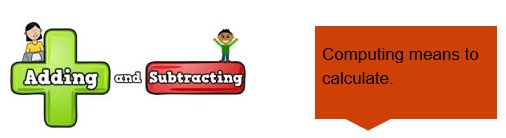This huge word consists of 2 parts computation which means to compute that is to calculate and other operations which mean methods or processes.

Hence the processes of performing certain operations to calculate values are known as computing operations.

• When we add or subtract numbers we calculate their addition and subtraction.

• And the process of doing so is called operation.

So the 4 computing operations are

2. Subtraction
3. Multiplication
4. Division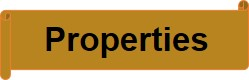The addition operation has 3 basic properties

1. 1. Commutative: It means sum is same even when the order of addends is changed.

Example 1- When we add 10 and 5, we get 15 that is 10+5=15 and when we add 5+10 we also get 15.

1. Associative: It says sum is independent of the grouping of addends.

Example 2- When we add 4 with 5 and then again add 4 to it so we can do it in two ways that is

4 + (5+4) = 4 + 9 =13 OR (4+5) + 4= 9 + 4 = 13.

1. Distributive: It says that we can multiply a sum by multiplying each addend separately and then add the products.

Example 3- When we multiply 5 with the sum of 4 and 5 we can do it in the following manner

4 x (5+4) = (4 x 5) + (4 x 4) = 20+ 16 = 36.

These properties are very useful for calculations of very large numbers and most important among them is distributive property.

Example 4- What is the multiplication of 7 and 14 and how can we makes it easier?

Solution: We can write it as 7 x (10+4) and by using distributive property we can write it as

7 x 14 = 7 x (10+4) = 7 x (4+10) = (7 x 10)+ (7 x 4)= 70+28= 98

Here it can be seen we have used commutative property also.

Subtraction

• The process of subtracting two numbers is called as subtraction.• Subtraction does not follow the associative, commutative and distributive property like addition.

Example 1: When we subtract 14 from 20 we get,

20 – 14 = 6 but when we subtract 20 from 14 we don’t get 6 and hence it proves that subtraction don’t follow the rule of commutative.

Example 2: When we subtract 2 from 8 and subtract 3 from it we get

(8-4) – 3 = 4 – 3 = 1 but on subtracting by using associative rule 8 – (4-3) =

8 – 1= 7 and hence it can be seen that it does follow the rule of associative.

Example 3:-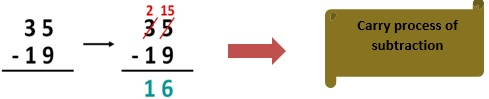Example 4: What will be the result when we subtract 1234 from 4321 that is 4321 – 1234 =?

Solution: Here we can see that on subtracting we get 3087 but if we use commutative property we get 1234-4321. So we can see that on subtracting we don’t get 3087 as answer and hence it also proves that subtraction does not follow commutative property.

Multiplication

• The process of multiplying two or more numbers is called multiplication.Like addition, multiplication also follows some basic properties which are:

1. Commutative: It says that the product of multiplication of two numbers is same even when the order of multiplicands is changed.

Example 1: When we multiply 5 with 6 we get 5 x 6 = 30 and on multiplying 6 with 5 we get 6 x 5 = 30 and hence it proves that multiplication also follows the commutative property.

1. Associative: It says that the product is independent of the grouping of the multiplicands.

Example 2: When we multiply 5 with 2 and then with 3 we get,

5 x (2 x 3) = 30 and it can be also done as (5 x 2) x 3 = 30 and hence it shows the associative property.

1. 3. Distributive: It says that we can multiply a sum by multiplying each addend separately and then add the products.

Example 3: When we multiply 7 with the sum of 4 and 3 we get

7 x (4+3) = (7 x 4) + (7 x 3) = 28 + 21 = 49.

Example 4: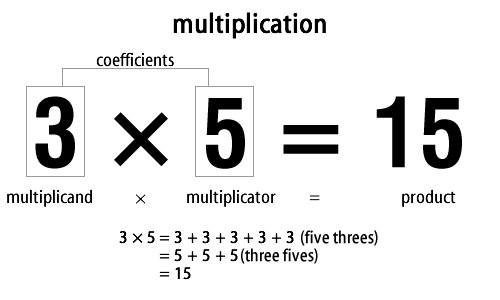• From the above example we get that we can add the no as same no. of time to get the result.

Division

• The process of dividing two numbers is called as division.
• Dividend:The number which is to be divided is called dividend.
• Divisor: Divisor is the number by which the dividend is divided.
• Quotient: The number of times the divisor divides the dividend is called the quotient.
• Remainder: The number which is left over after division is called the remainder.

Example 1: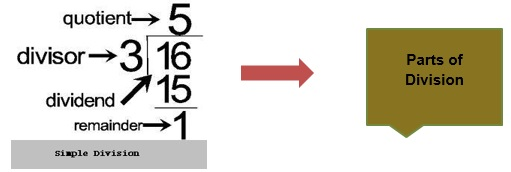Example 2: What we will get when 122÷6=?

Solution: Here the dividend is 122 and the divisor is 3.

And after dividing we get quotient as 20 and remainder as 2.

Practice Questions

Question 1: What will be the answer of 789+234=?

Question 2: What we will get when2345 is added to 1209?

Question 3: Write the answer of 897-349=?

Question 4: What we will get when 2331 is subtracted from 5649?

Question 5: What will be the product of 34 x 12=?

Question 6: What will you get on the multiplication of 21 with 7?

Question 7: State True or False-

2. 12 x 8 = 98
3. 2 + (4-7) = (2+4) - 7
4. Subtraction is distributive.

Recap

• Computation operations are the basic operations to calculate the values.
• Addition, subtraction, multiplication, division are the computation operations.
• Addition is the combining of two or more numbers to give sum of those numbers.
• Subtraction is removing number from another number to give final value.
• Subtraction and division does not follows the properties of associativity and commutative like addition and multiplication.
• Multiplication is addition of number same time as multiplied with.
• Division of two integers will not always give an integer.

## Quiz for Computing Operations

 Q.1 There are 6 rows of plants and each row has 4 plants. Find out the total number of plants in the garden. a) 12 b) 18 c) 24 d) 30
 Q.2 There are 10 plates on a dining table and each plate has 10 biscuits. How many biscuits are there on the dining table? a) 80 b) 90 c) 100 d) 110
 Q.3 A factory manufactured 945 car parts in a week and 1235 in the next week. Calculate the number of car parts manufactured in these two weeks. a) 2170 b) 2175 c) 2180 d) 2185
 Q.4 There are 26 units of speakers in a movie theater and every speaker uses 2 magnets in them. How many total magnets are there in the theater? a) 26 b) 52 c) 78 d) 104
 Q.5 A maximum of 12 men can sit on a bench. How many such benches are needed for 1524 men? a) 121 b) 123 c) 125 d) 127
 Q.6 There are 7846 students in a college, out of which 932 were absent today. How many students were present today? a) 6914 b) 5487 c) 3214 d) 5474
 Q.7 Gaurav is at level-4 in a video game and he has 3208 points. He needs 7398 more points to unlock level-5. At how many points does level-5 unlock? a) 5446 b) 10606 c) 4756 d) 7466
 Q.8 Shyam has 18 dining plates and he sells each plate for Rs. 20. How much does he earn after selling all of them? a) 180 b) 360 c) 540 d) 720
 Q.9 There are 156 water bottles in a park shop. The owner sells 23 of them in a day. How many bottles are left at the end of the day? a) 130 b) 133 c) 136 d) 139
 Q.10 A mobile phone costs Rs. 8000. How many such phones can be bought for Rs. 64000? a) 2 b) 4 c) 6 d) 8